Miscellaneous

Chapter 15 Class 11 Statistics (Term 1)
Serial order wise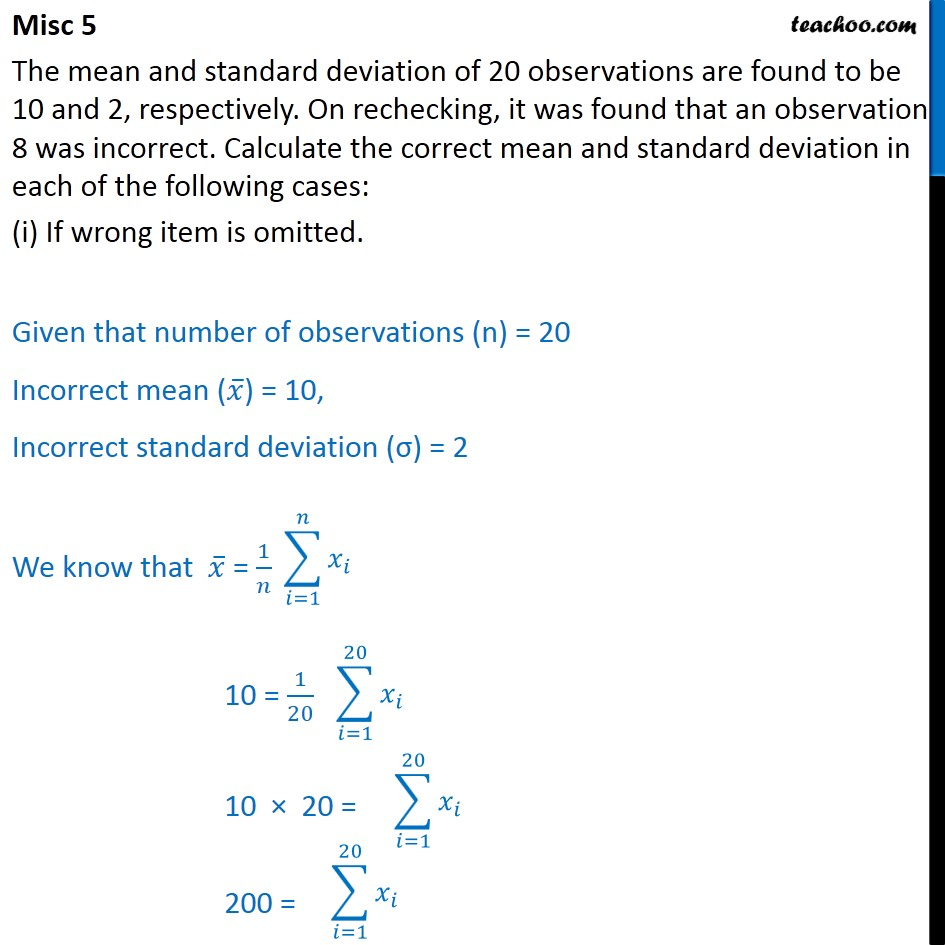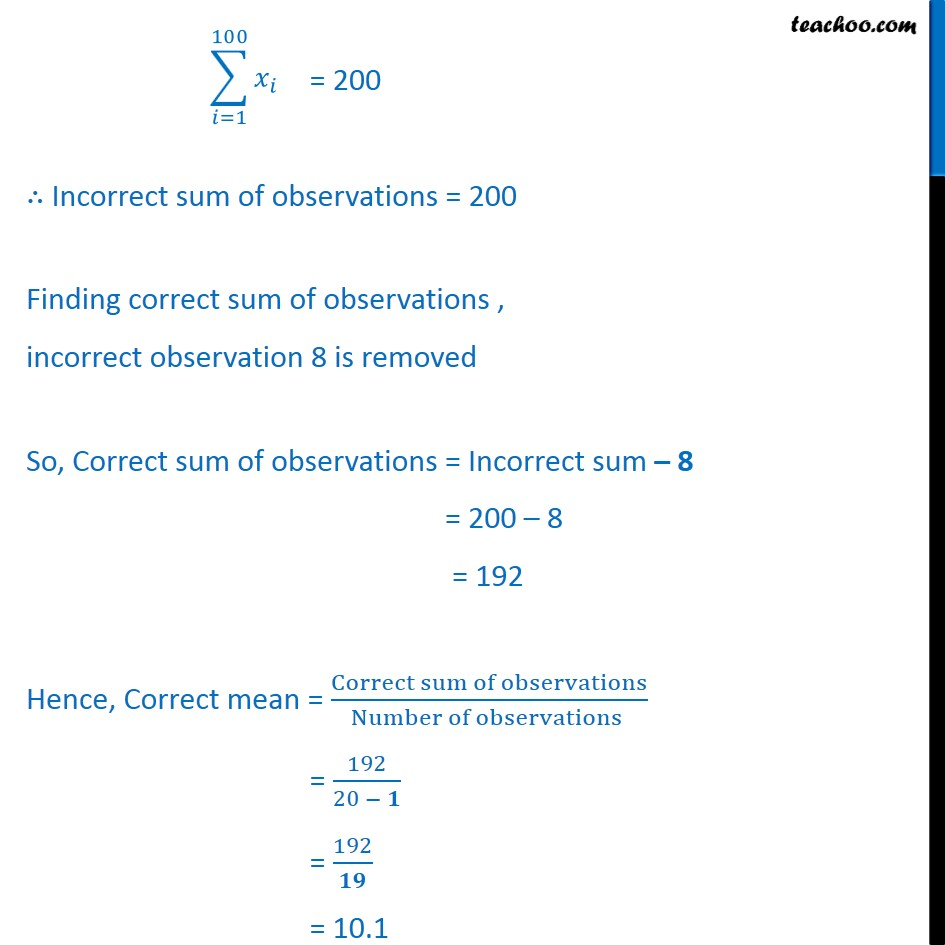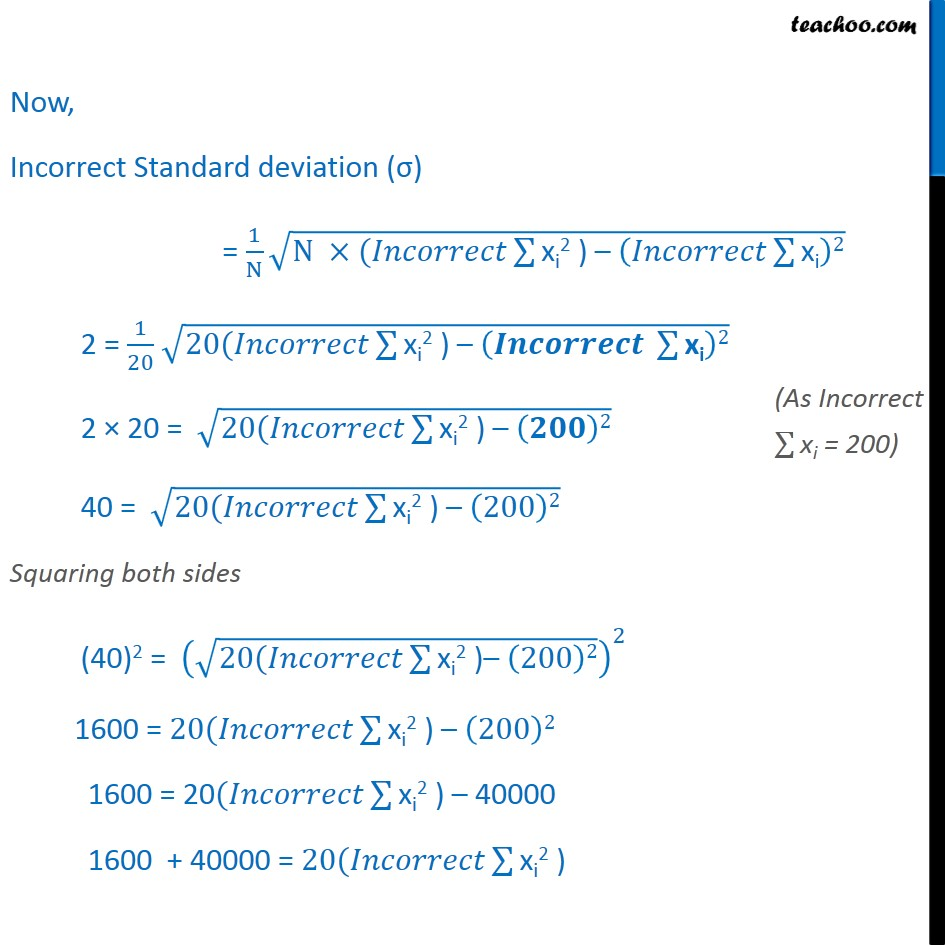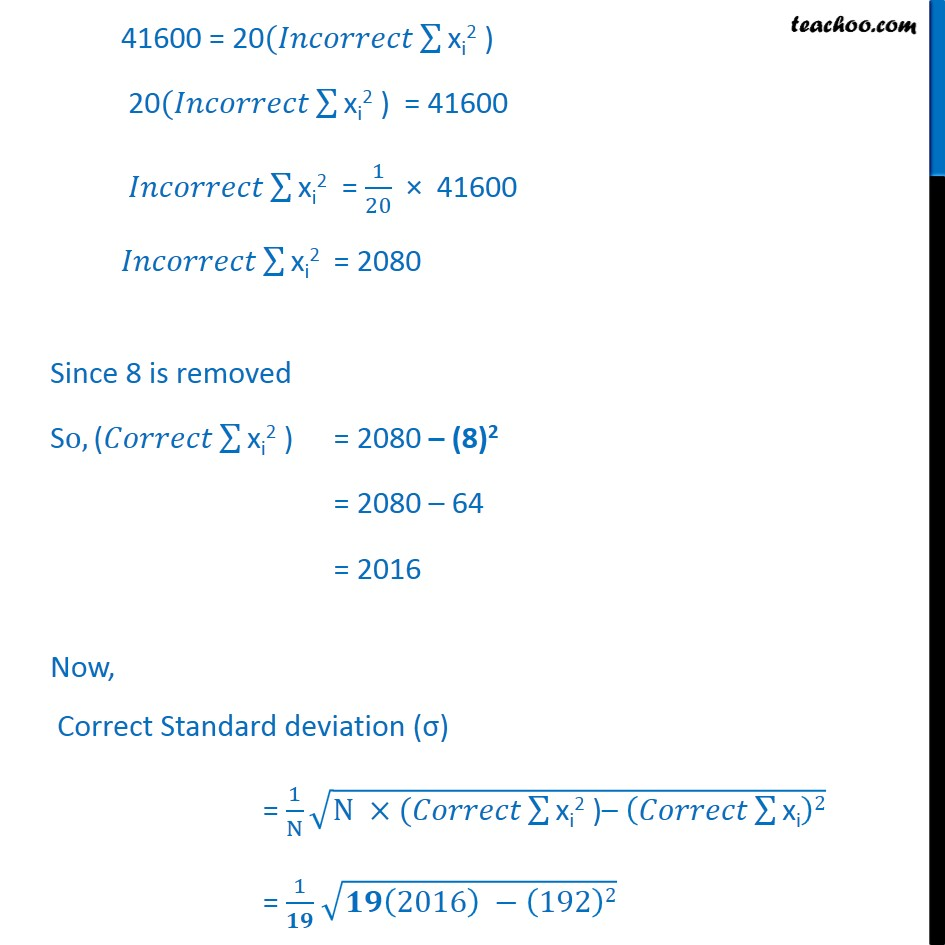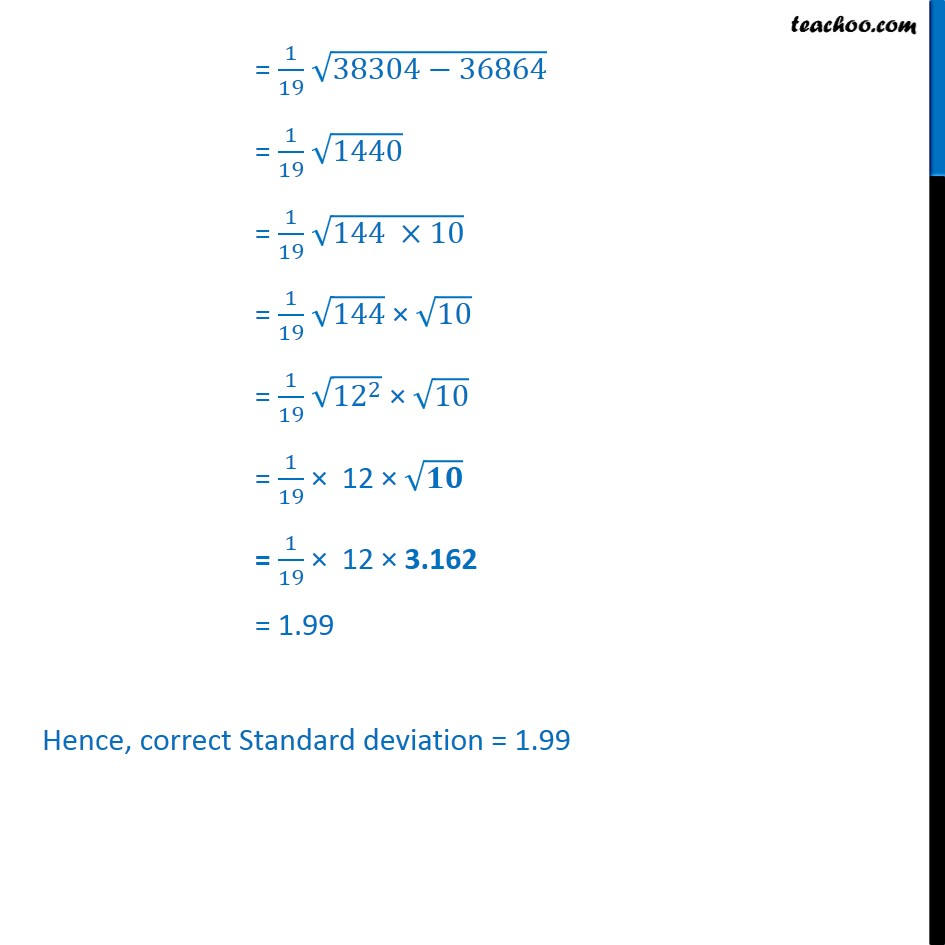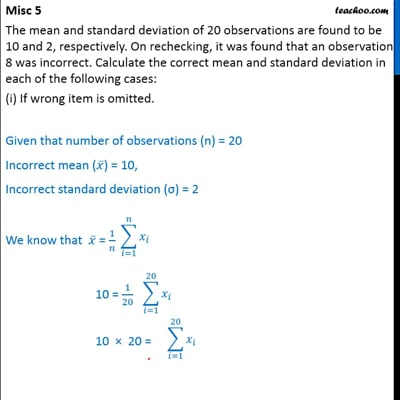This video is only available for Teachoo black users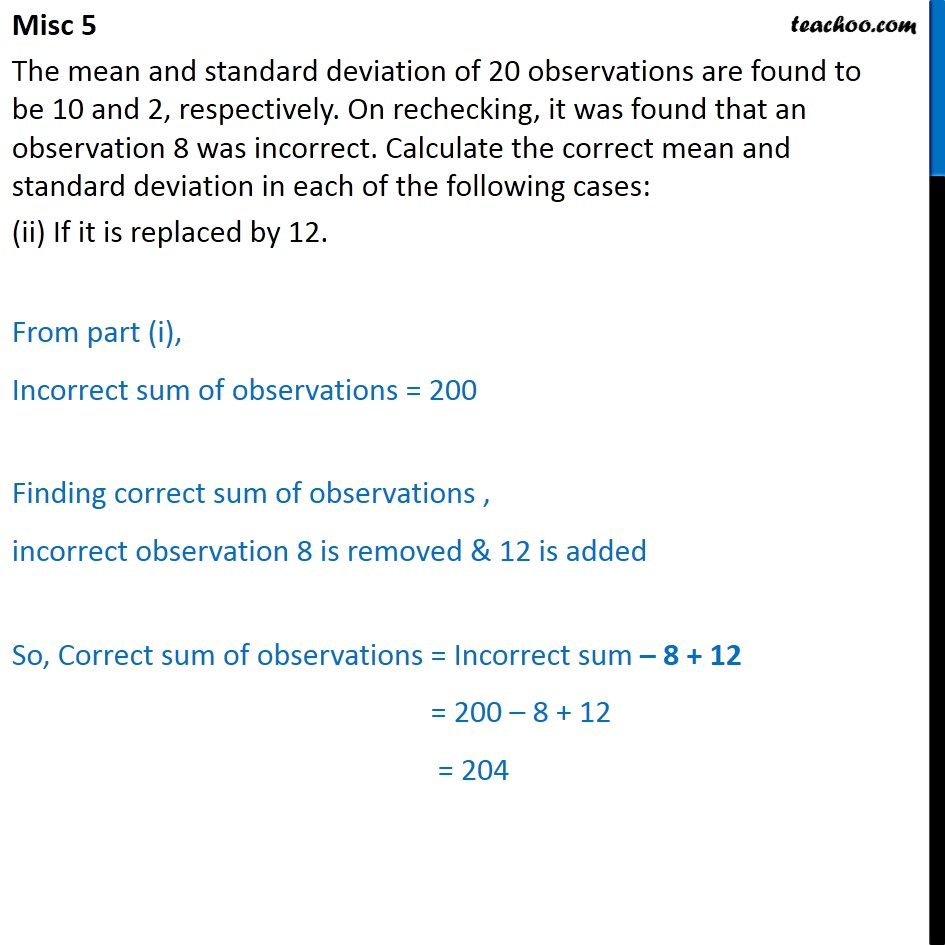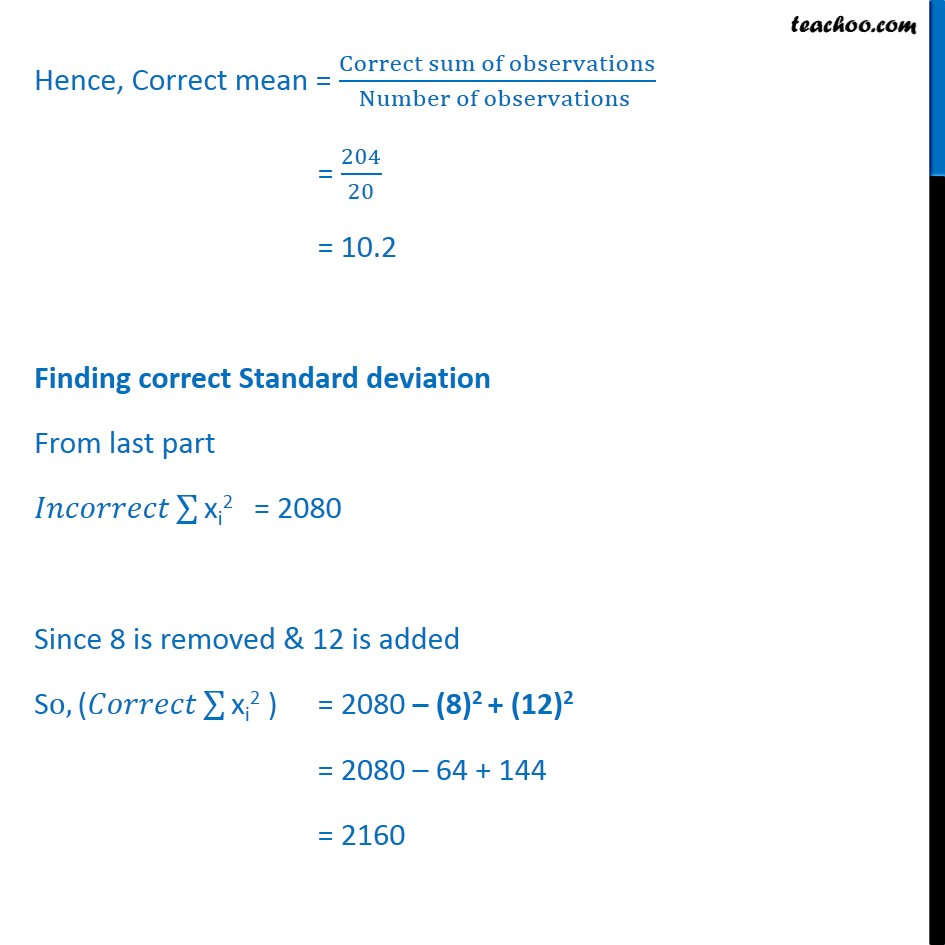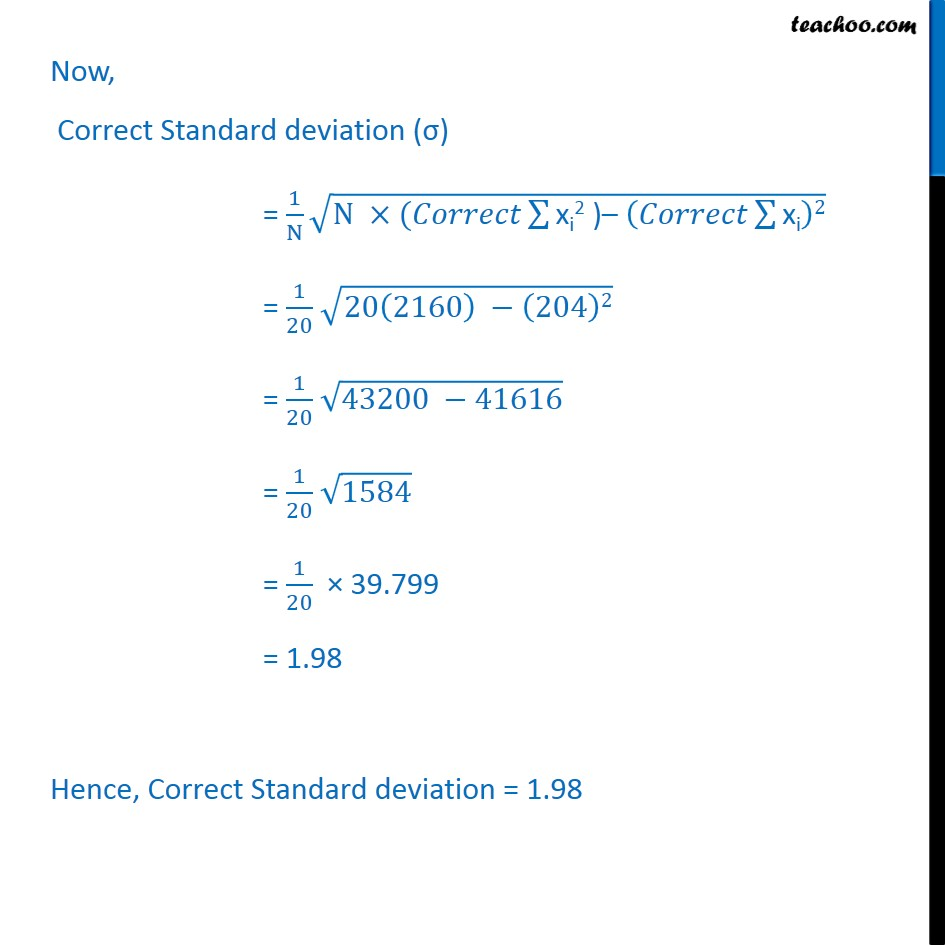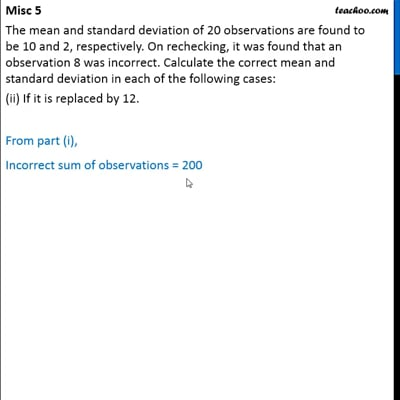This video is only available for Teachoo black users

### Transcript

Misc 5 The mean and standard deviation of 20 observations are found to be 10 and 2, respectively. On rechecking, it was found that an observation 8 was incorrect. Calculate the correct mean and standard deviation in each of the following cases: (i) If wrong item is omitted. Given that number of observations (n) = 20 Incorrect mean ( 𝑥﷯) = 10, Incorrect standard deviation (σ) = 2 We know that 𝑥﷯ = 1﷮𝑛﷯ 10 = 1﷮20﷯ 10 × 20 = 200 = = 200 ∴ Incorrect sum of observations = 200 Finding correct sum of observations , incorrect observation 8 is removed So, Correct sum of observations = Incorrect sum – 8 = 200 – 8 = 192 Hence, Correct mean = Correct sum of observations﷮Number of observations ﷯ = 192﷮20 − 𝟏﷯ = 192﷮𝟏𝟗﷯ = 10.1 Now, Incorrect Standard deviation (σ) = 1﷮N﷯ ﷮N ×(𝐼𝑛𝑐𝑜𝑟𝑟𝑒𝑐𝑡 ﷮﷮xi2 ) – 𝐼𝑛𝑐𝑜𝑟𝑟𝑒𝑐𝑡 ﷮﷮xi﷯﷯2﷯﷯ 2 = 1﷮20﷯ ﷮20(𝐼𝑛𝑐𝑜𝑟𝑟𝑒𝑐𝑡 ﷮﷮xi2 ) – 𝑰𝒏𝒄𝒐𝒓𝒓𝒆𝒄𝒕 ﷮﷮xi﷯﷯2﷯﷯ 2 × 20 = ﷮20(𝐼𝑛𝑐𝑜𝑟𝑟𝑒𝑐𝑡 ﷮﷮xi2 ) – 𝟐𝟎𝟎﷯2﷯﷯ 40 = ﷮20(𝐼𝑛𝑐𝑜𝑟𝑟𝑒𝑐𝑡 ﷮﷮xi2 ) – 200﷯2﷯﷯ Squaring both sides (40)2 = ﷮20(𝐼𝑛𝑐𝑜𝑟𝑟𝑒𝑐𝑡 ﷮﷮xi2 )– 200﷯2﷯﷯﷯﷮2﷯ 1600 = 20(𝐼𝑛𝑐𝑜𝑟𝑟𝑒𝑐𝑡 ﷮﷮xi2 ) – 200﷯2﷯ 1600 = 20(𝐼𝑛𝑐𝑜𝑟𝑟𝑒𝑐𝑡 ﷮﷮xi2 ) –﷯ 40000 1600 + 40000 = 20(𝐼𝑛𝑐𝑜𝑟𝑟𝑒𝑐𝑡 ﷮﷮xi2 ) ﷯ 41600 = 20(𝐼𝑛𝑐𝑜𝑟𝑟𝑒𝑐𝑡 ﷮﷮xi2 ) ﷯ 20(𝐼𝑛𝑐𝑜𝑟𝑟𝑒𝑐𝑡 ﷮﷮xi2 ) ﷯ = 41600 𝐼𝑛𝑐𝑜𝑟𝑟𝑒𝑐𝑡 ﷮﷮xi2 ﷯ = 1﷮20﷯ × 41600 𝐼𝑛𝑐𝑜𝑟𝑟𝑒𝑐𝑡 ﷮﷮xi2 ﷯ = 2080 Since 8 is removed So, (𝐶𝑜𝑟𝑟𝑒𝑐𝑡 ﷮﷮xi2 ) ﷯ = 2080 – (8)2 = 2080 – 64 = 2016 Now, Correct Standard deviation (σ) = 1﷮N﷯ ﷮N ×(𝐶𝑜𝑟𝑟𝑒𝑐𝑡 ﷮﷮xi2 )– 𝐶𝑜𝑟𝑟𝑒𝑐𝑡 ﷮﷮xi﷯﷯2﷯﷯ = 1﷮𝟏𝟗﷯ ﷮𝟏𝟗 2016﷯ − 192﷯2﷯ = 1﷮19﷯ ﷮38304−36864﷯ = 1﷮19﷯ ﷮1440﷯ = 1﷮19﷯ ﷮144 ×10﷯ = 1﷮19﷯ ﷮144﷯ × ﷮10﷯ = 1﷮19﷯ ﷮ 12﷮2﷯﷯ × ﷮10﷯ = 1﷮19﷯ × 12 × ﷮𝟏𝟎﷯ = 1﷮19﷯ × 12 × 3.162 = 1.99 Hence, correct Standard deviation = 1.99 Misc 5 The mean and standard deviation of 20 observations are found to be 10 and 2, respectively. On rechecking, it was found that an observation 8 was incorrect. Calculate the correct mean and standard deviation in each of the following cases: (ii) If it is replaced by 12. From part (i), Incorrect sum of observations = 200 Finding correct sum of observations , incorrect observation 8 is removed & 12 is added So, Correct sum of observations = Incorrect sum – 8 + 12 = 200 – 8 + 12 = 204 Hence, Correct mean = Correct sum of observations﷮Number of observations ﷯ = 204﷮20﷯ = 10.2 Finding correct Standard deviation From last part 𝐼𝑛𝑐𝑜𝑟𝑟𝑒𝑐𝑡 ﷮﷮xi2 ﷯ = 2080 Since 8 is removed & 12 is added So, (𝐶𝑜𝑟𝑟𝑒𝑐𝑡 ﷮﷮xi2 ) ﷯ = 2080 – (8)2 + (12)2 = 2080 – 64 + 144 = 2160 Now, Correct Standard deviation (σ) = 1﷮N﷯ ﷮N ×(𝐶𝑜𝑟𝑟𝑒𝑐𝑡 ﷮﷮xi2 )– 𝐶𝑜𝑟𝑟𝑒𝑐𝑡 ﷮﷮xi﷯﷯2﷯﷯ = 1﷮20﷯ ﷮20 2160﷯ − 204﷯2﷯ = 1﷮20﷯ ﷮43200 −41616﷯ = 1﷮20﷯ ﷮1584﷯ = 1﷮20﷯ × 39.799 = 1.98 Hence, Correct Standard deviation = 1.98